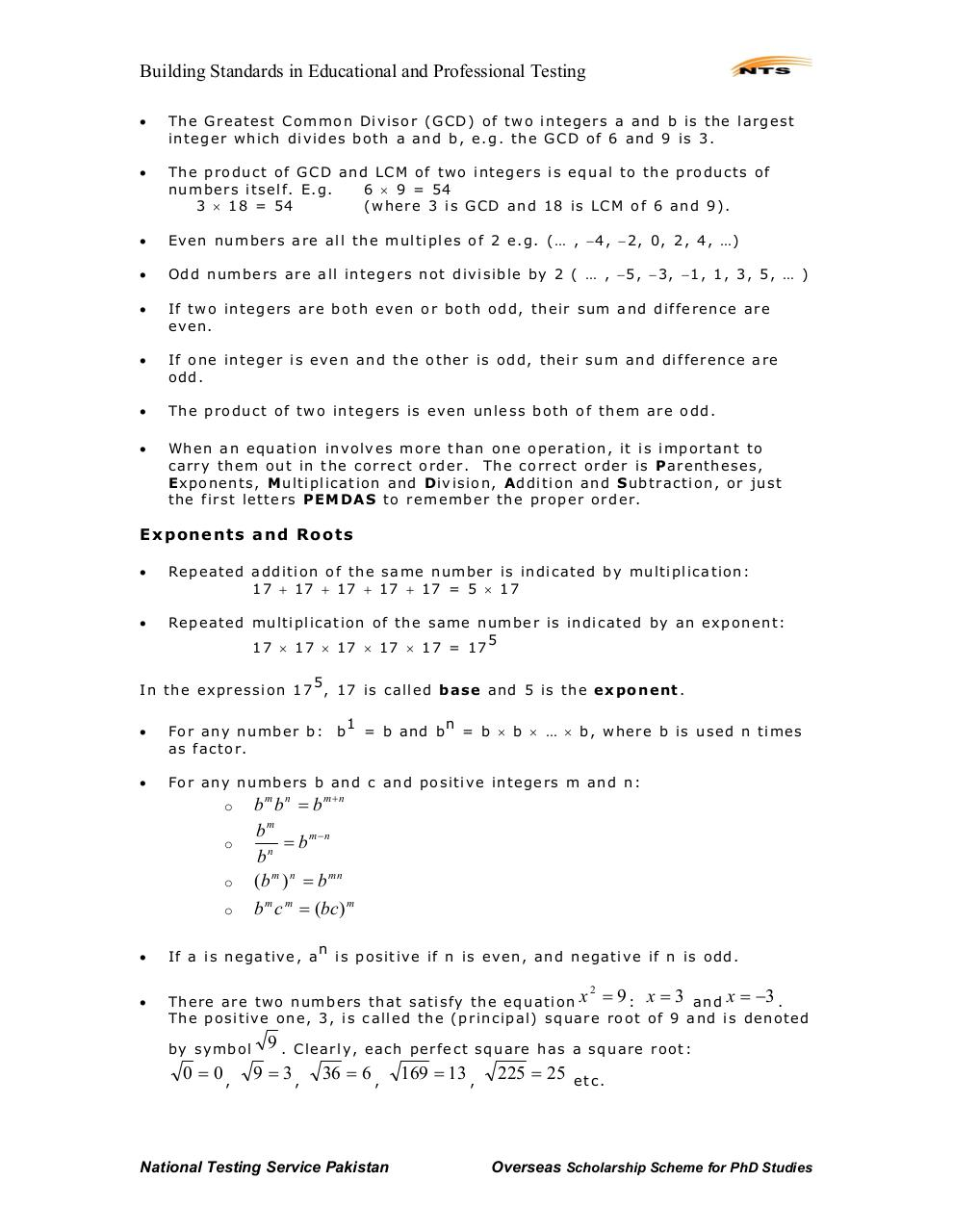# NTS Book for GAT General.pdfPage 1...3 4 567142

#### Text preview

Building Standards in Educational and Professional Testing

The Greatest Common Di vi sor (GCD) of tw o integers a and b is th e largest
i n teger which di vi des both a and b, e. g. the GCD of 6 and 9 i s 3.

The produc t of GCD an d LCM of two i n tegers i s equ al to th e prod u cts of
n u m bers i tsel f . E. g.
6 × 9 = 54
3 × 18 = 54
(where 3 i s GCD and 18 i s LCM of 6 and 9).

Even numbers are al l the mul ti ples of 2 e. g. (… , −4, −2, 0, 2, 4, …)

Odd numbe rs are all integers no t di vi si bl e by 2 ( … , −5, −3, −1, 1, 3, 5, … )

If two i n tegers are bo th even or both odd, t h ei r sum and di fference are
even.

If one i n teger i s even and the other i s odd, thei r sum and di fference are
odd.

The produc t of two i n tegers i s even unl ess both of them are odd.

When an equati on invol v es more than one operati on, i t i s i m portant to
carry them out i n the correct order. The correct order i s P arentheses,
Exponents, Mu l ti pli cati on an d Div i si on , Add i ti on an d Subtracti on, or just
the fi rst l etters PEM D AS to remember th e proper or der.

E x p o n e n ts a nd R o o ts

Repe ated a ddi ti on of the same number i s indi cated by mul ti pli cation:
17 + 17 + 1 7 + 17 + 17 = 5 × 17

Repe ated mul ti pli cation of the same number i s i n di cated by an e x ponent:
5
17 × 17 × 17 × 17 × 17 = 17

5
In the expressi on 17 , 17 i s called base and 5 i s the e x p o n e n t.

For any numbers b an d c and po si tive integers m and n:

o
o
o

= b and b

n

For any number b:
as factor.

o

b

1

= b × b × … × b, where b i s used n ti mes

b m b n = b m+n
bm
= b m−n
n
b
(b m ) n = b mn
b m c m = (bc) m
n

If a is negative, a

i s posi ti v e i f n i s ev en , an d n egati v e i f n i s odd.

There are t w o numbers that sati sf y the equati on x = 9 : x = 3 a n d x = −3 .
The posi ti ve one, 3, is call ed the (pri nci pal ) square root of 9 and i s denoted
2

by symbol

9.

0 = 0,

9 = 3,

Cl earl y, each perfect square has a square root:

36 = 6 ,

National Testing Service Pakistan

169 = 13 ,

225 = 25

etc.

Overseas Scholarship Scheme for PhD Studies